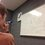# The Golden Ratio: Another Link to the Fibonacci's

Here is the previous post concerning the Golden Ratio. For a collection of all the posts concerning the Golden Ratio, click #GoldenRatio below.

It's been a while since I've last posted. I apologize. School has been busy, as well as the recent math olympiad contests here in the US. So, to refresh your memory of the Golden Ratio, remember that it is the positive solution to the following equation:

$\phi^2 = \phi + 1$

and

$\phi = \frac{1+\sqrt{5}}{2}$

Also remember that the Fibonacci numbers are described by the recurrence relation

$F_1 = 1, \, F_2 = 1, \, F_{n+1} = F_n + F_{n-1}$

Now, the golden ratio is tied into the Fibonacci numbers in this way: the ratios of successive Fibonacci numbers approaches the golden ratio as $n$ approaches infinity. However, there is even more connecting the golden ratio to the Fibonacci numbers, as we will explore here.

Notice the first equation above. What happens if I multiply both sides by $\phi$? $\phi^2$? $\phi^{n-1}$? Let's see with the last one:

$\phi^{n+1} = \phi^n + \phi^{n-1}$

Aha! This is exactly like that Fibonacci number formula, but with powers of the golden ratio instead. Since they look very much the same, they also act very much the same. For instance, what if I wanted to find $F_7$, the seventh Fibonacci number? Well, I would create the sequence:

$1,\, 1,\, 2,\, 3,\, 5,\, 8,\, 13$

and see that the seventh term is $F_7=13$ I can do the same thing with the golden ratio, but based off of different starting values: $\phi^0 = 1$, $\phi^1 = \phi$. Then our sequence (starting at $\phi^0$) becomes:

$1,\, \phi,\, 1+\phi,\, 1+2\phi,\, 2+3\phi,\, 3+5\phi,\, 5+8\phi,\, 8+13\phi$

Thus, $\phi^7 = 8 + 13\phi$. Any integral power of $\phi$ can be written in $a + b\phi$ form, and you should see the pattern for the numbers $a$ and $b$.

PROBLEM 1: Prove that $\phi^n = F_{n-1} + F_{n} \phi$ for all $n\in \mathbb{Z}$

In fact, any sequence $G_n$ that follows the Fibonacci recurrence relation will follow this more general formula that $G_n = F_{n-1} G_0 + F_{n} G_1$. Now, using this fact with the golden ratio, we can expand that to the other root of the first equation above, which is $\frac{-1}{\phi}$ (try and check that yourself). Since this value satisfies the same initial formula, it will satisfy our deduced formula, namely

$\left( \frac{-1}{\phi}\right)^n = F_{n-1} + F_{n}\left( \frac{-1}{\phi}\right)$

Subtracting this from the equation in Problem 1, we get

$\phi^n - \left( \frac{-1}{\phi}\right)^n = F_n \left(\phi-\left( \frac{-1}{\phi}\right)\right)$

$\Rightarrow F_n = \frac{\phi^n - \left( \frac{-1}{\phi}\right)^n}{\phi-\left( \frac{-1}{\phi}\right)}$

or

$F_n = \frac{\phi^n - (-\phi)^{-n}}{\sqrt{5}}$

This is known as Binet's formula, although the result was known to Euler and de Moivre before. Binet developed a more general form of the formula, which we may discuss later.

Note the implications of this formula. We know longer have to calculate $F_1,\, F_2,\,\cdots,\, F_{99}$ to find $F_{100}$, for if we have a calculator, we can simply use this formula. Also, because $|(-\phi)^{-n}|<1$ for all positive values of $n$, we can abbreviate the formula to

$F_n = \left[\frac{\phi^n}{\sqrt{5}}\right]$

where $[a]$ represents the nearest integer to $a$.

Test your knowledge with these questions:

Problem 1

Problem 2

More to comeNote by Bob Krueger
7 years, 4 months ago

This discussion board is a place to discuss our Daily Challenges and the math and science related to those challenges. Explanations are more than just a solution — they should explain the steps and thinking strategies that you used to obtain the solution. Comments should further the discussion of math and science.

When posting on Brilliant:

• Use the emojis to react to an explanation, whether you're congratulating a job well done , or just really confused .
• Ask specific questions about the challenge or the steps in somebody's explanation. Well-posed questions can add a lot to the discussion, but posting "I don't understand!" doesn't help anyone.
• Try to contribute something new to the discussion, whether it is an extension, generalization or other idea related to the challenge.

MarkdownAppears as
*italics* or _italics_ italics
**bold** or __bold__ bold
- bulleted- list
• bulleted
• list
1. numbered2. list
1. numbered
2. list
Note: you must add a full line of space before and after lists for them to show up correctly
paragraph 1paragraph 2

paragraph 1

paragraph 2

[example link](https://brilliant.org)example link
> This is a quote
This is a quote
    # I indented these lines
# 4 spaces, and now they show
# up as a code block.

print "hello world"
# I indented these lines
# 4 spaces, and now they show
# up as a code block.

print "hello world"
MathAppears as
Remember to wrap math in $$ ... $$ or $ ... $ to ensure proper formatting.
2 \times 3 $2 \times 3$
2^{34} $2^{34}$
a_{i-1} $a_{i-1}$
\frac{2}{3} $\frac{2}{3}$
\sqrt{2} $\sqrt{2}$
\sum_{i=1}^3 $\sum_{i=1}^3$
\sin \theta $\sin \theta$
\boxed{123} $\boxed{123}$

Sort by:

Please start a post like this for teaching calculus, I don't know what it is.....

- 7 years, 4 months ago

This is an awesome thread. Please continue with the notes and problems.

- 7 years, 4 months ago

Thanks Bob for helping many people learn things.........Please keep the noble great awesome work up!

- 7 years, 4 months ago

Nice Bob continue this type of thread.

- 7 years, 4 months ago

awesome post bob, thanks

- 7 years, 4 months ago